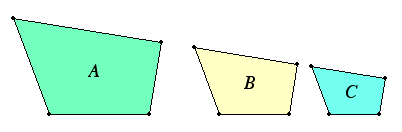# Proposition 21

Figures which are similar to the same rectilinear figure are also similar to one another.

Let each of the rectilinear figures A and B be similar to C.I say that A is also similar to B.

VI.Def.1

Since A is similar to C, it is equiangular with it and has the sides about the equal angles proportional.

Again, since B is similar to C, it is equiangular with it and has the sides about the equal angles proportional.

V.11

Therefore each of the figures A and B is equiangular with C and with C has the sides about the equal angles proportional, therefore A is similar to B.

Therefore, figures which are similar to the same rectilinear figure are also similar to one another.

Q.E.D.

## Guide

This proposition is used in the proofs of propositions VI.24, VI.28, and VI.29. It also would have been useful in the proof of VI.8.